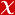## Rotary and percussive drilling prediction using regression analysis

INTERNATIONAL JOURNAL OF ROCK MECHANICS AND MINING SCIENCES, vol.36, no.7, pp.981-989, 1999 (Journal Indexed in SCI)• Publication Type: Article / Article
• Volume: 36 Issue: 7
• Publication Date: 1999
• Doi Number: 10.1016/s0148-9062(99)00050-9
• Title of Journal : INTERNATIONAL JOURNAL OF ROCK MECHANICS AND MINING SCIENCES
• Page Numbers: pp.981-989

#### Abstract

It has recently been conjectured that the eigenvalues $\lambda$ of the Dirac operator on a closed Riemannian spin manifold $M$ of dimension $n\ge 3$ can be estimated from below by the total scalar curvature: $$\lambda^2 \ge \frac{n}{4(n-1)} \cdot \frac{\int_M S}{vol(M)}.$$ We show by example that such an estimate is impossible.